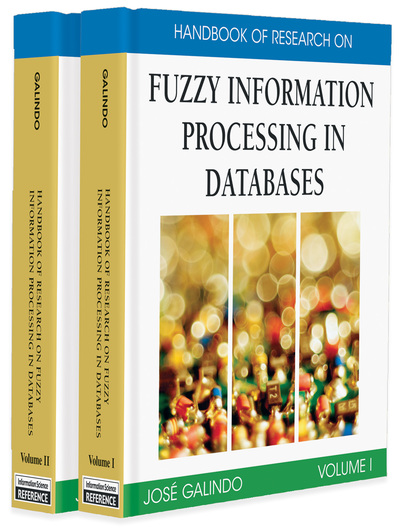# Introduction and Trends to Fuzzy Logic and Fuzzy Databases

José Galindo (University of Málaga, Spain)
DOI: 10.4018/978-1-59904-853-6.ch001
Available
\$37.50
No Current Special Offers

## Abstract

This chapter presents an introduction to fuzzy logic and to fuzzy databases. With regard to the first topic, we have introduced the main concepts in this field to facilitate the understanding of the rest of the chapters to novel readers in fuzzy subjects. With respect to the fuzzy databases, this chapter gives a list of six research topics in this fuzzy area. All these topics are briefly commented on, and we include references to books, papers, and even to other chapters of this handbook, where we can find some interesting reviews about different subjects and new approaches with different goals. Finally, we give a historic summary of some fuzzy models, and we conclude with some future trends in this scientific area.

## Key Terms in this Chapter

Soft Computing: Computational techniques in computer science and some engineering disciplines, which attempt to study, model, and analyze very complex phenomena: those for which more conventional methods have not yielded low cost, analytic, and complete solutions. Earlier computational approaches could model and precisely analyze only relatively simple systems. More complex systems arising in biology, medicine, the humanities, management sciences, artificial intelligence, machine learning, and similar fields often remained intractable to conventional mathematical and analytical methods. Soft computing techniques include: fuzzy systems (FS), neural networks (NN), evolutionary computation (EC), probabilistic reasoning (PR), and other ideas (chaos theory, etc.). Soft computing techniques often complement each other.

Fuzzy Database: If a regular or classical database is a structured collection of information (records or data) stored in a computer, a fuzzy database is a database which is able to deal with uncertain or incomplete information using fuzzy logic. There are many forms of adding flexibility in fuzzy databases. The simplest technique is to add a fuzzy membership degree to each record, that is, an attribute in the range [0,1]. However, there are other kinds of databases allowing fuzzy values to be stored in fuzzy attributes using fuzzy sets, possibility distributions, or fuzzy degrees associated to some attributes and with different meanings (membership degree, importance degree, fulfillment degree, etc.). Of course, fuzzy databases should allow fuzzy queries using fuzzy or nonfuzzy data and there are some languages that allow this kind of queries, like FSQL or SQLf. In synthesis, the research in fuzzy databases includes the following areas: flexible querying in classical or fuzzy databases, extending classical data models in order to achieve fuzzy databases (fuzzy relational databases, fuzzy object-oriented databases, etc.), fuzzy conceptual modeling, fuzzy data mining techniques, and applications of these advances in real databases.

T-conorm or S-norm: Function s establishing a generic model for the operation of union with fuzzy sets. These functions must comply with certain basic properties: commutative, associative, monotonicity, and border conditions (x s 0 = x, and x s 1 = 1). The most typical is the maximum function, but other widely accepted s-norms exist (Table 2).

Fuzzy Implication: Function computing the fulfillment degree of a rule expressed by IF X THEN Y, where the antecedent and the consequent are fuzzy. These functions must comply with certain basic properties and the most typical is the Kleene-Dienes implication, based on the classical implication definition (x?y = ¬x ? y), using the Zadeh’s negation and the maximum s-norm, but other fuzzy implication functions exist (Table 3).

T-norm: Function t establishing a generic model for the operation of intersection with fuzzy sets. These functions must comply with certain basic properties: commutative, associative, monotonicity, and border conditions (x t 0 = 0, and x t 1 = x). The most typical is the minimum function, but there exists other t-norms widely accepted (Table 1).

Fuzzy Quantifiers: Expressions allowing us to express fuzzy quantities or proportions in order to provide an approximate idea of the number of elements of a subset fulfilling a certain condition or of the proportion of this number in relation to the total number of possible elements. Fuzzy quantifiers can be absolute or relative. Absolute quantifiers express quantities over the total number of elements of a particular set, stating whether this number is, for example, “much more than 10,” “close to 100,” “a great number of,” and so forth. Relative quantifiers express measurements over the total number of elements, which fulfill a certain condition depending on the total number of possible elements. This type of quantifier is used in expressions such as “the majority” or “most,” “the minority,” “little of,” “about half of,” and so on.

Fuzzy Attribute: In a database context, a fuzzy attribute is an attribute of a row or object in a database, with a fuzzy datatype, which allows storing fuzzy information. Sometimes, if a classic attribute allows fuzzy queries, then it is also called fuzzy attribute, because it has only some of the fuzzy attribute characteristics.

Fuzzy Logic: Fuzzy logic is derived from fuzzy set theory by Zadeh (1965), dealing with reasoning that is approximate rather than precisely deduced from classical predicate logic. It can be thought of as the application side of fuzzy set theory dealing with well thought out real world expert values for a complex problem.

Possibility Theory: This theory is based on the idea that we can evaluate the possibility of a determinate variable X being (or belonging to) a determinate set or event A. Here, fuzzy sets are called possibility distributions and instead of measuring the membership degrees, they measure the possibility degrees. All the tools and properties defined for fuzzy sets are also applicable to possibility distributions.

## Complete Chapter List

Search this Book:
Reset